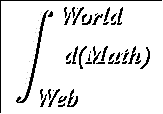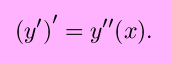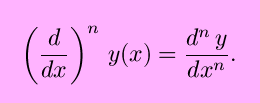#The Notation of Differentiation

Suggested Prerequesites: The definition of the derivative

Often the most confusing thing for a student introduced to differentiation is the notation associated with it. Here an attempt will be made to introduce as many types of notation as possible.A derivative is always the derivative of a function with respect to a variable. When we write the definition of the derivative aswe mean the derivative of the function f(x) with respect to the variable x.One type of notation for derivatives is sometimes called prime notation. The function f´(x), which would be read ``f-prime of x'', means the derivative of f(x) with respect to x. If we say y = f(x), then y´ (read ``y-prime'') = f´(x). This is even sometimes taken as far as to write things such as, for y = x4 + 3x (for example), y´ =  (x4 + 3x)´.Higher order derivatives in prime notation are represented by increasing the number of primes. For example, the second derivative of y with respect to x would be written asBeyond the second or third derivative, all those primes get messy, so often the order of the derivative is instead writen as a roman superscript in parenthesis, so that the ninth derivative of f(x) with respect to x is written as f(9)(x) or f(ix)(x).A second type of notation for derivatives is sometimes called operator notation. The operator Dx is applied to a function in order to perform differentiation. Then, the derivative of f(x) = y with respect to x can be written as Dxy (read ``D -- sub -- x of y'') or as Dxf(x (read ``D -- sub x -- of -- f(x)'').Higher order derivatives are written by adding a superscript to Dx, so that, for example the third derivative of y =  (x2+sin(x)) with respect to x would be written asAnother commonly used notation was developed by Leibnitz and is accordingly called Leibnitz notation. With this notation, if y = f(x), then the derivative of y with respect to x can be written as(his is read as ``dy -- dx'', but not ``dy minus dx'' or sometimes ``dy over dx''). Since y = f(x), we can also writeThis notation suggests that perhaps derivatives can be treated like fractions, which is true in limited ways in some circumstances. (For example with the chain rule.) This is also called differential notation, where dy and dx are differentials. This notation becomes very useful when dealing with differential equations.A variation of Leibnitz's differential notation is written instead aswhich resembles the above operator notation, with (d/dx as the operator).Higher order derivatives using leibnitz notation can be written asThe exponents may seem to be in strange places in the second form, but it makes sense if you look at the first form.

So, those are the most commonly used notations for differentiation. It's possible that there exist other, obscure notations used by a some, but these obscure forms won't be included here. It's helpful to be familiar with the different notations.
When is a function differentiable?

watko@mit.edu
Last updated August 24, 1998 <\body>